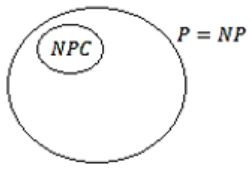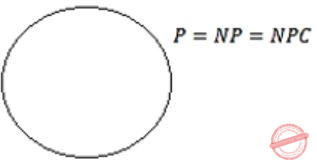## P-NP

 Question 1

Consider two decision problems Q1, Q2 such that Q1 reduces in polynomial time to 3-SAT and 3 -SAT reduces in polynomial time to Q2. Then which one of following is consistent with the above statement?

 A Q1 is in NP, Q2 in NP hard B Q1 is in NP, Q2 is NP hard C Both Q1 and Q2 are in NP D Both Q1 and Q2 are NP hard
Theory-of-Computation       P-NP       GATE 2015 [Set-2]
Question 1 Explanation:
3-SAT ⇒ NPC problem
Q1≤p 3-SAT≤p Q2 ≤p ≤p hence → Q1 is in NP
but Q2 is not given in NP
Hence Q2 is in NP-Hard.
 Question 2

Suppose a polynomial time algorithm is discovered that correctly computes the largest clique in a given graph. In this scenario, which one of the following represents the correct Venn diagram of the complexity classes P, NP and NP Complete (NPC)?

 ABCDAlgorithms       P-NP       GATE 2014 [Set-1]
Question 2 Explanation:
The most important open question in complexity theory is whether the P = NP, which asks whether polynomial time algorithms actually exist for NP-complete and all NP problems (since a problem “C” is in NP-complete, iff C is in NP and every problem in NP is reducible to C in polynomial time). In the given question it is given that some polynomial time algorithm exists which computes the largest clique problem in the given graph which is known NP-complete problem. Hence P=NP=NP-Complete.
 Question 3

Assuming P ≠ NP, which of the following is TRUE?

 A NP-complete = NP B NP-complete ∩ P = ∅ C NP-hard = NP D P = NP-complete
Algorithms       P-NP       GATE 2012
Question 3 Explanation:
Note: Out of syllabus.
The definition of NP-complete is,
A decision problem p is NP-complete if:
1. p is in NP, and
2. Every problem in NP is reducible to p in polynomial time.
It is given that assume P ≠ NP , hence NP-complete ∩ P = ∅ .
This is due to the fact that, if NP-complete ∩ P ≠ ∅ i.e. there are some problem (lets say problem P1) which is in P and in NP-complete also, then it means that P1 (NP-complete problem) can be solved in polynomial time also (as it is also in P class) and this implies that every NP problem can be solve in polynomial time, as we can convert every NP problem into NP-complete problem in polynomial time.
Which means that we can convert every NP problem to P1 and solve in polynomial time and hence P = NP, which is contradiction to the given assumption that P ≠ NP.
 Question 4

Let πA be a problem that belongs to the class NP. Then which one of the following is TRUE?

 A There is no polynomial time algorithm for πA. B If πA can be solved deterministically in polynomial time,then P = NP. C If πA is NP-hard, then it is NP-complete. D πA may be undecidable.
Algorithms        P-NP       GATE 2009
Question 4 Explanation:
Note: Out of syllabus.
 Question 5

The subset-sum problem is defined as follows: Given a set S of n positive integers and a positive integer W, determine whether there is a subset of S Whose elements sum to

An algorithm Q solves this problem in O(nW) time. Which of the following statements is false?

 A Q solves the subset-sum problem in polynomial time when the input is encoded in unary B Q solves the subset-sum problem in polynomial time when the input is encoded in binary C The subset sum problem belongs to the class NP D The subset sum problem is NP-hard
Algorithms       P-NP       GATE 2008
Question 5 Explanation:
Note: Out of syllabus.
 Question 6

Let S be an NP-complete problem and Q and R be two other problems not known to be in NP. Q is polynomial time reducible to S and S is polynomial-time reducible to R. Which one of the following statements is true?

 A R is NP-complete B R is NP-hard C Q is NP-complete D Q is NP-hard
Algorithms        P-NP       GATE 2006
Question 6 Explanation:
NP-Hard- if it can be solved in polynomial time then all NP-Complete can be solved in polynomial time. If NP Hard problem is reducible to another problem in Polynomial Time, then that problem is also NP Hard which means every NP problem can be reduced to this problem in Polynomial Time.
 Question 7

Let SHAM3 be the problem of finding a Hamiltonian cycle in a graph G = (V,E) with |V| divisible by 3 and DHAM3 be the problem of determining if a Hamiltonian cycle exists in such graphs. Which one of the following is true?

 A Both DHAM3 and SHAM3 are NP-hard B SHAM3 is NP-hard, but DHAM3 is not C DHAM3 is NP-hard, but SHAM3 is not D Neither DHAM3 nor SHAM3 is NP-hard
Algorithms        P-NP       GATE 2006
Question 7 Explanation:
Whether to finding a Hamiltonian cycles exist (or) not is a NP Hard problem. So both DHAM3 and SHAM3 are NP-hard.
 Question 8

Consider the following two problems on undirected graphs:

α: Given G(V,E), does G have an independent set of size |V|-4?
β: Given G(V,E), does G have an independent set of size 5?

Which one of the following is TRUE?

 A α is in P and β is NP-complete B α is NP-complete and β is in P C Both α and β are NP-complete D Both α and β are in P
Algorithms        P-NP       GATE 2005
Question 8 Explanation:
Graph independent set decision problem is belongs to NP-complete.
There are 8 questions to complete.

Register Now